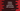# Python Module : Python tutorial 18## Python Module :

A python module is simply a python file ( .py file ) with definitions and statements. The file name is the module name. After creating a module, we can use it any other module using “import” command.

Module is useful for re-usability of the code. You can write one function in one module and import this module in different modules and reuse that function .

## Built in modules :

We can use built-in modules using the same “import” syntax. e.g. “math” is a built in python module, “sqrt” is one function defined in “math”. To use “sqrt”, we should first import “math” to our program.

``````import math
print math.sqrt(4)``````

This will print 4.0

## Find details of a python module :

As we have seen above, “math” is a system module and “sqrt” is one of its function. How to get all functions available in “math” ? use dir( modulename )_ :

``````import math
print dir(math)``````

It will print :

``['__doc__', '__file__', '__name__', '__package__', 'acos', 'acosh', 'asin', 'asinh', 'atan', 'atan2', 'atanh', 'ceil', 'copysign', 'cos', 'cosh', 'degrees', 'e', 'erf', 'erfc', 'exp', 'expm1', 'fabs', 'factorial', 'floor', 'fmod', 'frexp', 'fsum', 'gamma', 'hypot', 'isinf', 'isnan', 'ldexp', 'lgamma', 'log', 'log10', 'log1p', 'modf', 'pi', 'pow', 'radians', 'sin', 'sinh', 'sqrt', 'tan', 'tanh', 'trunc']``

Similarly, to get details of a function, we can use “help” .

``````import math
print help(math.sqrt)``````

It will print :

``````Help on built-in function sqrt in module math:

sqrt(...)
sqrt(x)

Return the square root of x.
(END)``````

## User defined python module :

Create one file mymodule.py as below :

``````def boo():
return "inside boo function.."

def booboo():
return "inside booboo function.."``````

Now create one temp.py file in the same folder and import “mymodule” as :

``````import mymodule

print mymodule.boo()
print mymodule.booboo()``````

If you execute_ temp.py_, It will print the following :

``````inside boo function..
inside booboo function..``````

We can also import the name of the functions using from…import statement as below :

``````from mymodule import boo,booboo

print boo()
print booboo()``````

It will print same result as shown in the above example.

Or we can import all function names using “ * “ :

``````from mymodule import *

print boo()
print booboo()``````

## Importing a module by renaming it :

We can import a module and rename it using import….as statement :

``````import mymodule as m

print m.boo()
print m.booboo()``````

## Import one module multiple times :

If we import a module multiple times, it is imported only for once. If we include “import” more than one time , then also it will be executed only one time. Let’s change mymodule.py as below :

``````def boo():
return "inside boo function.."

def booboo():
return "inside booboo function.."

Now change temp.py as below :

``````import mymodule as m
import mymodule as m2

print m.boo()
print m.booboo()``````

run temp.py and you will get the following output :

``````mymodule loaded
inside boo function..
inside booboo function..``````

i.e._ mymodule.py_ is run only for once .